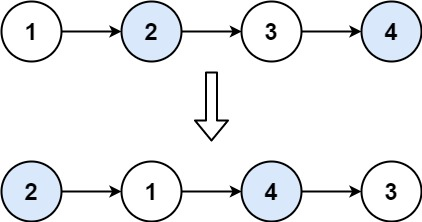### LeetCode• ㊗️
• 大家
• offer
• 多多！

## Problem

Given a linked list, swap every two adjacent nodes and return its head. You must solve the problem without modifying the values in the list’s nodes (i.e., only nodes themselves may be changed.)

Example 1:Input: head = [1,2,3,4]
Output: [2,1,4,3]


Example 2:

Input: head = []
Output: []


Example 3:

Input: head = 
Output: 


Constraints:

• The number of nodes in the list is in the range [0, 100].
• 0 <= Node.val <= 100

## Code

class Solution {

ListNode dummy = new ListNode(0);
ListNode curr = dummy;

while(curr.next != null && curr.next.next != null){
ListNode first = curr.next;
ListNode second = curr.next.next;

curr.next = second;
first.next = second.next;
second.next = first;

curr = curr.next.next;
}

return dummy.next;
}
}